MAHARASHTRA

XII (12) HSC

XI (11) FYJC
X (10) SSC

### Relations and Functions, Ex. No. 1.2, 10th New Syllabus 2019 - 2020.

Relations and Functions,
Ex. No. 1.2

(1)  Let A = {1, 2, 3, 7} and B = {3, 0, –1, 7}, which of the following are relation from A to B ?
(i)  R1 = {(2, 1), (7, 1)}
(ii) R2 =  {(–1,1)}
(iii) R3 = {(2, –1), (7, 7), (1, 3)}
(iv) R4= {(7,–1), (0,3), (3,3), (0,7)}
(i)  R1 = {(2, 1), (7, 1)}
Solution :
Cartesian product of A and B
A x B  = {(1, 3) (1, 0) (1, -1) (1, 7) (2, 3) (2, 0) (2, -1) (2, 7)(3, 3) (3, 0) (3   , -1)(3, 7)(7, 3)(7, 0)(7, -1)(7, 7)}
Note : We don't have to list out all these things. In order to understand, we have listed out.
R1 ∈ (2, 1) ∉ A x B. Hence R1 is not a relation.
(ii) R2 =  {(–1,1)}
Solution :
(-1, 1) is a element of R2, but in Cartesian product, we have (1, -1).
(1, -1) (-1, 1). Hence R2 is not a relation.
(iii) R3 = {(2, –1), (7, 7), (1, 3)}
Solution :
R3 is a subset of A x B, Hence it is a relation from A to B.
(iv) R4= {(7,–1), (0,3), (3,3), (0,7)}
Solution :
The element (7,–1) ∈ A x B
But (0,3), (3,3), (0,7) ∉ A x B
Hence it is not a relation.

(2)  Let A = {1, 2, 3, 4,..., 45} and R be the relation defined as “is square of ” on A. Write R as a subset of A x A. Also, find the domain and range of R.
Solution :
A = {1, 2, 3, 4,..., 45}
Let "A" be a set which contains some of elements of A
A = {1, 2, 3, 4, 5, 6}
Now, we have to write the other set of elements from A. The elements in this set denotes the square value of previous set.
Square values of set A  = {1, 4, 9, 16, 25, 36}
A x A  = {(1, 1)(1,4)(1, 9)(1, 16) (1, 25)  (1, 36)..................}
Domain = {1, 2, 3, 4, 5, 6}
Range  = {1, 4, 9, 16, 25, 36}

(3)  A Relation R is given by the set {(x, y) /y = x + 3, x ∈  {0, 1, 2, 3, 4, 5}}. Determine its domain and range.
Solution :
Given that :
y = x + 3

 y = x + 3 x = 0 y = 0 + 3 y = 3 y = x + 3 x = 1 y = 1 + 3 y = 4 y = x + 3 x = 2 y = 2 + 3 y = 5 y = x + 3 x = 3 y = 3 + 3 y = 6 y = x + 3 x = 4 y = 4 + 3 y = 7 y = x + 3 x = 5 y = 5 + 3 y = 8

R = {(0, 3) (1, 4) (2, 5) (3, 6) (4, 7) (5, 8)}
Domain of R = {0, 1, 2, 3, 4, 5}
Range of R = {3, 4, 5, 6, 7, 8}

(4)  Represent each of the given relations by (a) an arrow diagram, (b) a graph and (c) a set in roster form, wherever possible.
(i) {(x, y)| x = 2y, x {2, 3, 4, 5}, y {1, 2, 3, 4}   Solution
Solution :
Given that :
x = 2y
y = x/2

 if x = 2 y = 2/2 y = 1 f x = 3 y = 3/2 if x = 4 y = 4/2 y = 2 if x = 5 y = 5/2

Hence the required relation is {(2, 1) (3, 3/2) (4, 2) (5, 5/2)}
(i)  Arrow diagram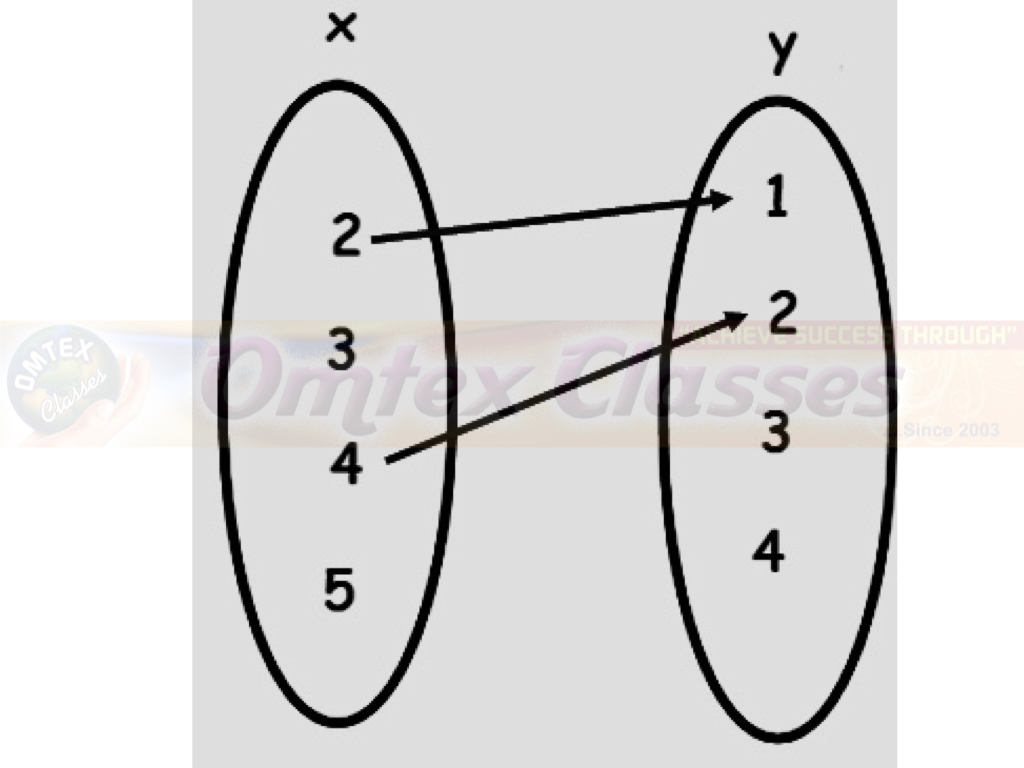(ii)  A graph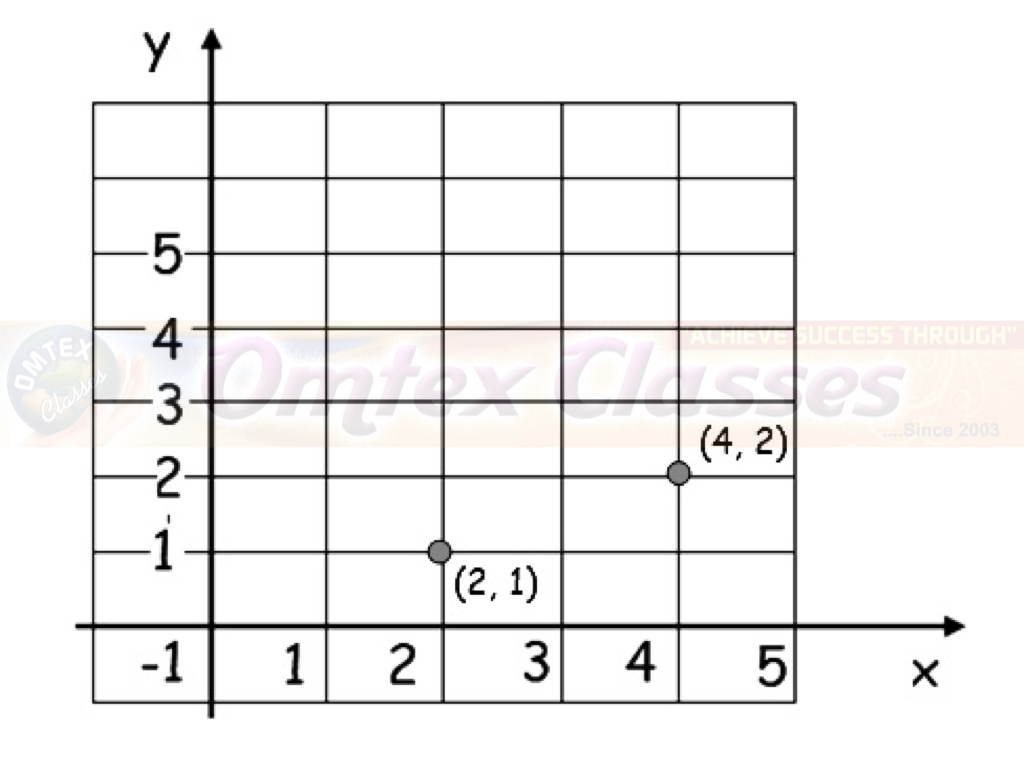(iii)  in roster form
= {(2, 1) (4, 2)}

(ii) {(x, y) | y = x + 3, x, y are natural numbers < 10}    Solution

Solution :
Given that :
y = x + 3

 if x = 1 y = 4 if x = 2 y = 5 if x = 3 y = 6 if x = 4 y = 7 if x = 5 y = 8 if x = 6 y = 9

R = {(1, 4) (2, 5) (3, 6) (4, 7) (5, 8) (6, 9)}
An arrow diagram :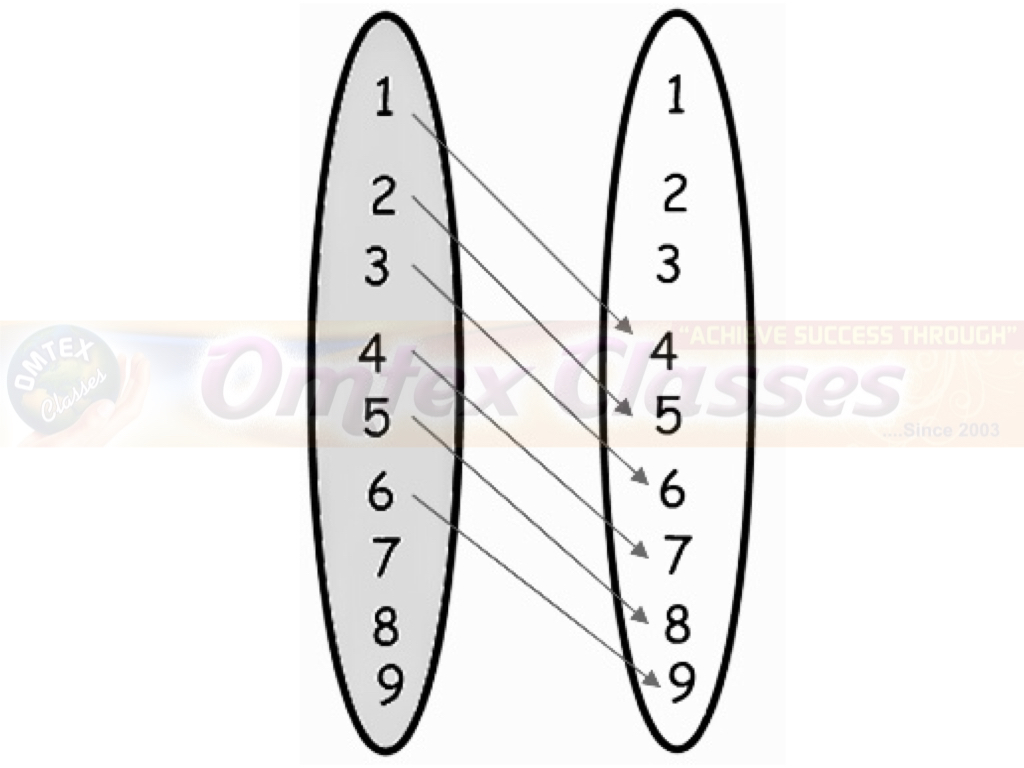(ii)  A graph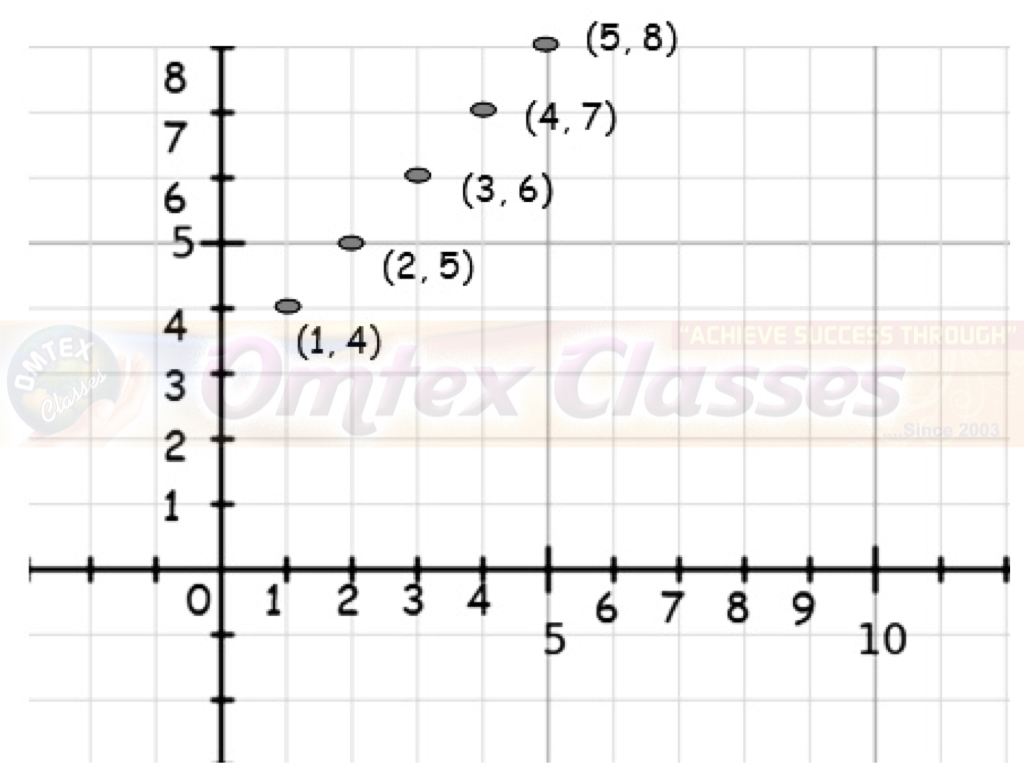In Roster form :
= {(1, 4) (2, 5) (3, 6) (4, 7) (5, 8) (6, 9)}

(5)  A company has four categories of employees given by Assistants (A), Clerks (C), Managers (M) and an Executive Officer (E). The company provide ₹10,000, ₹25,000, ₹50,000 and ₹1,00,000 as salaries to the people who work in the categories A, C, M and E respectively. If A1, A2, A3, A4and A5 were Assistants; C1, C2, C3, C4 were Clerks; M1, M2, M3 were managers and E1, E2 were Executive officers and if the relation R is defined by xRy, where x is the salary given to person y, express the relation R through an ordered pair and an arrow diagram.
Solution :
"x" be the salary given to person
"y" be the set of employees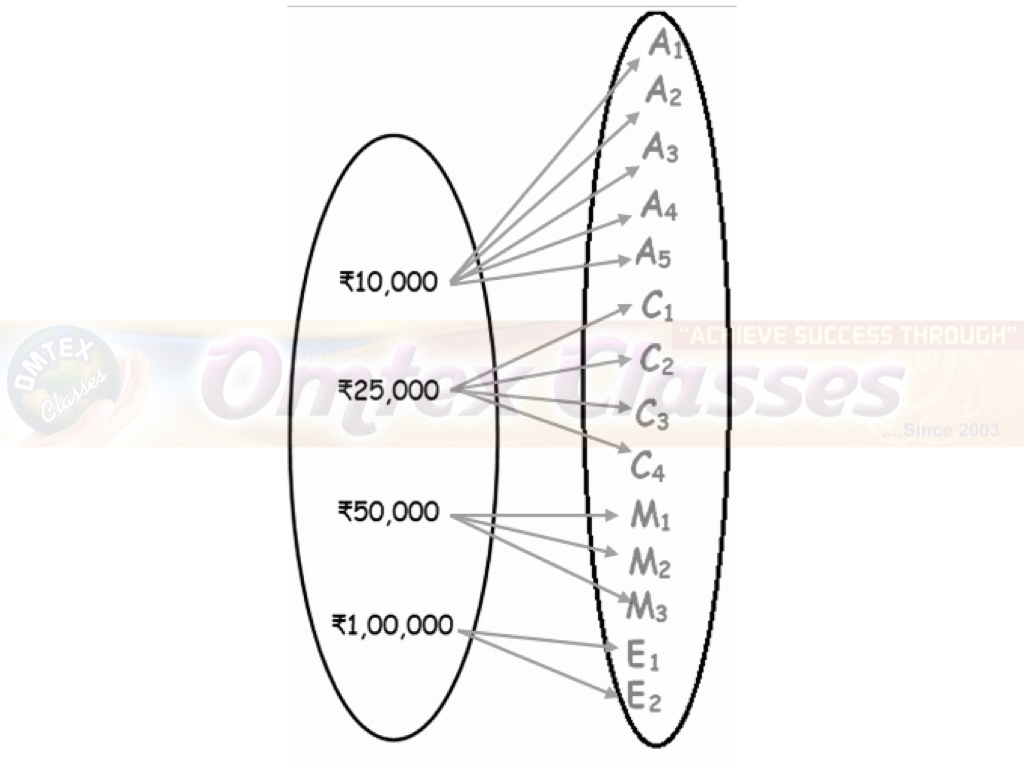## PDF FILE TO YOUR EMAIL IMMEDIATELY PURCHASE NOTES & PAPER SOLUTION. @ Rs. 50/- each (GST extra)

SUBJECTS

HINDI ENTIRE PAPER SOLUTION

MARATHI PAPER SOLUTION
SSC MATHS I PAPER SOLUTION
SSC MATHS II PAPER SOLUTION
SSC SCIENCE I PAPER SOLUTION
SSC SCIENCE II PAPER SOLUTION
SSC ENGLISH PAPER SOLUTION
SSC & HSC ENGLISH WRITING SKILL
HSC ACCOUNTS NOTES
HSC OCM NOTES
HSC ECONOMICS NOTES
HSC SECRETARIAL PRACTICE NOTES

2019 Board Paper Solution

HSC ENGLISH SET A 2019 21st February, 2019

HSC ENGLISH SET B 2019 21st February, 2019

HSC ENGLISH SET C 2019 21st February, 2019

HSC ENGLISH SET D 2019 21st February, 2019

SECRETARIAL PRACTICE (S.P) 2019 25th February, 2019

HSC XII PHYSICS 2019 25th February, 2019

CHEMISTRY XII HSC SOLUTION 27th, February, 2019

OCM PAPER SOLUTION 2019 27th, February, 2019

HSC MATHS PAPER SOLUTION COMMERCE, 2nd March, 2019

HSC MATHS PAPER SOLUTION SCIENCE 2nd, March, 2019

SSC ENGLISH STD 10 5TH MARCH, 2019.

HSC XII ACCOUNTS 2019 6th March, 2019

HSC XII BIOLOGY 2019 6TH March, 2019

HSC XII ECONOMICS 9Th March 2019

SSC Maths I March 2019 Solution 10th Standard11th, March, 2019

SSC MATHS II MARCH 2019 SOLUTION 10TH STD.13th March, 2019

SSC SCIENCE I MARCH 2019 SOLUTION 10TH STD. 15th March, 2019.

SSC SCIENCE II MARCH 2019 SOLUTION 10TH STD. 18th March, 2019.

SSC SOCIAL SCIENCE I MARCH 2019 SOLUTION20th March, 2019

SSC SOCIAL SCIENCE II MARCH 2019 SOLUTION, 22nd March, 2019

XII CBSE - BOARD - MARCH - 2019 ENGLISH - QP + SOLUTIONS, 2nd March, 2019

HSC Maharashtra Board Papers 2020

(Std 12th English Medium)

HSC ECONOMICS MARCH 2020

HSC OCM MARCH 2020

HSC ACCOUNTS MARCH 2020

HSC S.P. MARCH 2020

HSC ENGLISH MARCH 2020

HSC HINDI MARCH 2020

HSC MARATHI MARCH 2020

HSC MATHS MARCH 2020

SSC Maharashtra Board Papers 2020

(Std 10th English Medium)

English MARCH 2020

HindI MARCH 2020

Hindi (Composite) MARCH 2020

Marathi MARCH 2020

Mathematics (Paper 1) MARCH 2020

Mathematics (Paper 2) MARCH 2020

Sanskrit MARCH 2020

Important-formula

THANKS